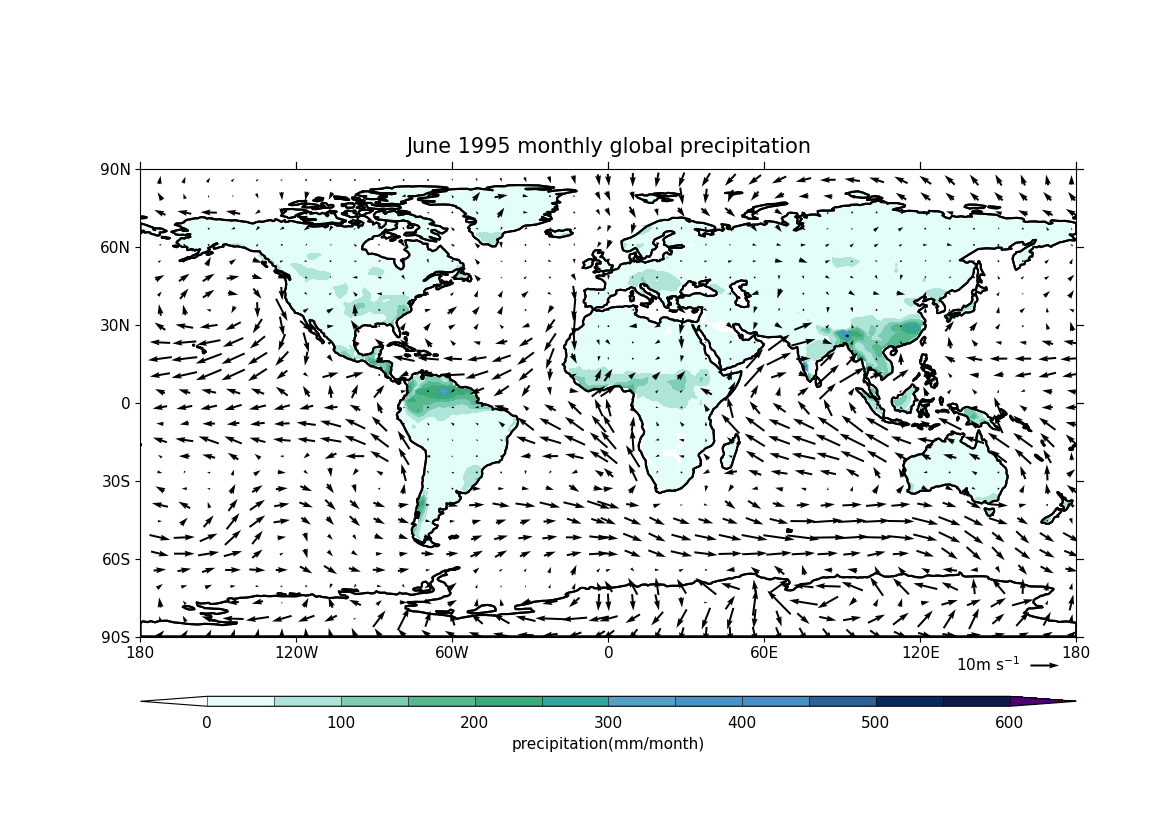# Plotting wind vectors overlaid on precipitation data¶

In this recipe we will plot wind vectors, derived from northward and eastward wind components, over precipitation data.

1. Import cf-python and cf-plot:

import cfplot as cfp

import cf


f1 = cf.read("~/recipes/northward.nc")
print(f1)

[<CF Field: northward_wind(time(1980), latitude(144), longitude(192)) m s-1>]

f2 = cf.read("~/recipes/eastward.nc")
print(f2)

[<CF Field: eastward_wind(time(1980), latitude(144), longitude(192)) m s-1>]

f3 = cf.read("~/recipes/monthly_precipitation.nc")
print(f3)

[<CF Field: long_name=precipitation(long_name=time(1452), latitude(144), longitude(192)) mm/month>]

1. Select wind vectors and precipitation data by index and look at their contents:

v = f1
print(v)

Field: northward_wind (ncvar%vas)
---------------------------------
Data            : northward_wind(time(1980), latitude(144), longitude(192)) m s-1
Cell methods    : area: time(1980): mean
Dimension coords: time(1980) = [1850-01-16 00:00:00, ..., 2014-12-16 00:00:00] 360_day
: latitude(144) = [-89.375, ..., 89.375] degrees_north
: longitude(192) = [0.0, ..., 358.125] degrees_east
: height(1) = [10.0] m

u = f2
print(u)

Field: eastward_wind (ncvar%uas)
--------------------------------
Data            : eastward_wind(time(1980), latitude(144), longitude(192)) m s-1
Cell methods    : area: time(1980): mean
Dimension coords: time(1980) = [1850-01-16 00:00:00, ..., 2014-12-16 00:00:00] 360_day
: latitude(144) = [-89.375, ..., 89.375] degrees_north
: longitude(192) = [0.0, ..., 358.125] degrees_east
: height(1) = [10.0] m

pre = f3
print(pre)

Field: long_name=precipitation (ncvar%pre)
------------------------------------------
Data            : long_name=precipitation(long_name=time(1452), latitude(144), longitude(192)) mm/month
Dimension coords: long_name=time(1452) = [1901-01-16 00:00:00, ..., 2021-12-16 00:00:00] gregorian
: latitude(144) = [-89.375, ..., 89.375] degrees_north
: longitude(192) = [0.0, ..., 358.125] degrees_east

1. Plot the wind vectors on top of precipitation data for June 1995 by creating a subspace with a date-time object and using cfplot.con. Here cfplot.gopen is used to define the parts of the plot area, which is closed by cfplot.gclose; cfplot.cscale is used to choose one of the colour maps amongst many available; cfplot.levs is used to set the contour levels for precipitation data; and cfplot.vect is used to plot the wind vectors for June 1995:

june_95 = cf.year(1995) & cf.month(6)
cfp.gopen()
cfp.cscale("precip4_11lev")
cfp.levs(step=100)
cfp.con(
pre.subspace(T=june_95),
lines=False,
title="June 1995 monthly global precipitation",
)
cfp.vect(
u=u.subspace(T=june_95),
v=v.subspace(T=june_95),
key_length=10,
scale=35,
stride=5,
)
cfp.gclose()Total running time of the script: ( 0 minutes 6.238 seconds)

Gallery generated by Sphinx-Gallery